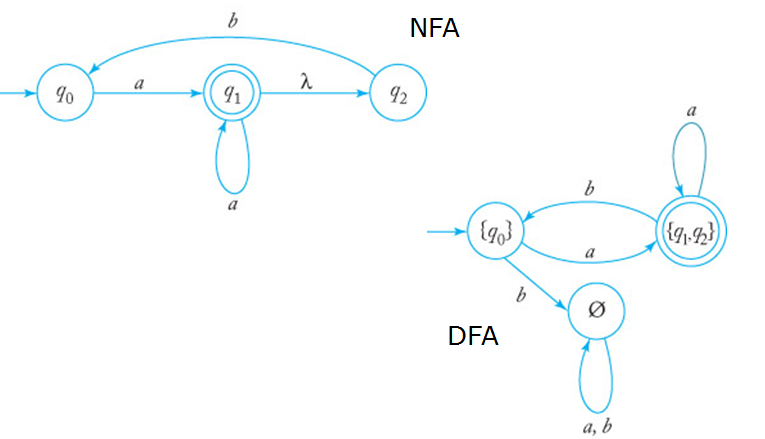Finite State MachineFinite state automata or finite state machines , is a simple mathematical model, with a finite set of discrete inputs, and is received through an input according to the order determined by a finite set of states . Automatic machine may have a finite set of output. If so, the unit will automatically generate a series of outputs to reflect the sequence of input. Currency exchange machines, elevators, vending machines, and the garage door opener is an example based on the model of the machine. In many areas of computer science, finite state is very useful design models, including the vocabulary compiler scans, in order to calculate the agreed definitions, and switching circuits.

Here is a Power Point Presentation, that explains all the required basic knowledge to understand this topic.

.

## Finite State Machine:

### Finite Automata

Share.1.